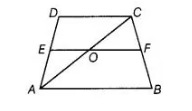# E is the mid-point of the side AD of the trapezium`
Question:

E is the mid-point of the side AD of the trapezium ABCD with AB || DC. A line through E drawn parallel to AB intersects BC at F. Show thatf is the mid-

point of BC.

Thinking Process

Use the mid-point theorem i.e., the line segment joining the mid-points of two sides of a triangle is parallel to the third side and half of it. Further

shown the required result.

Solution:

Given ABCD is a trapezium in which AB || DC and EF||AB|| CD.

Construction Join, the diagonal AC which intersects EF at O.

To show F is the mid-point of BC.Proof Now, in ΔADC, E is the mid-point of AD and OE || CD. Thus, by mid-point theorem, O is mid-point of AC.

Now, in ΔCBA, 0 is the mid-point of AC and OF || AB.

So, by mid-point theorem, F is the mid-point of BC.